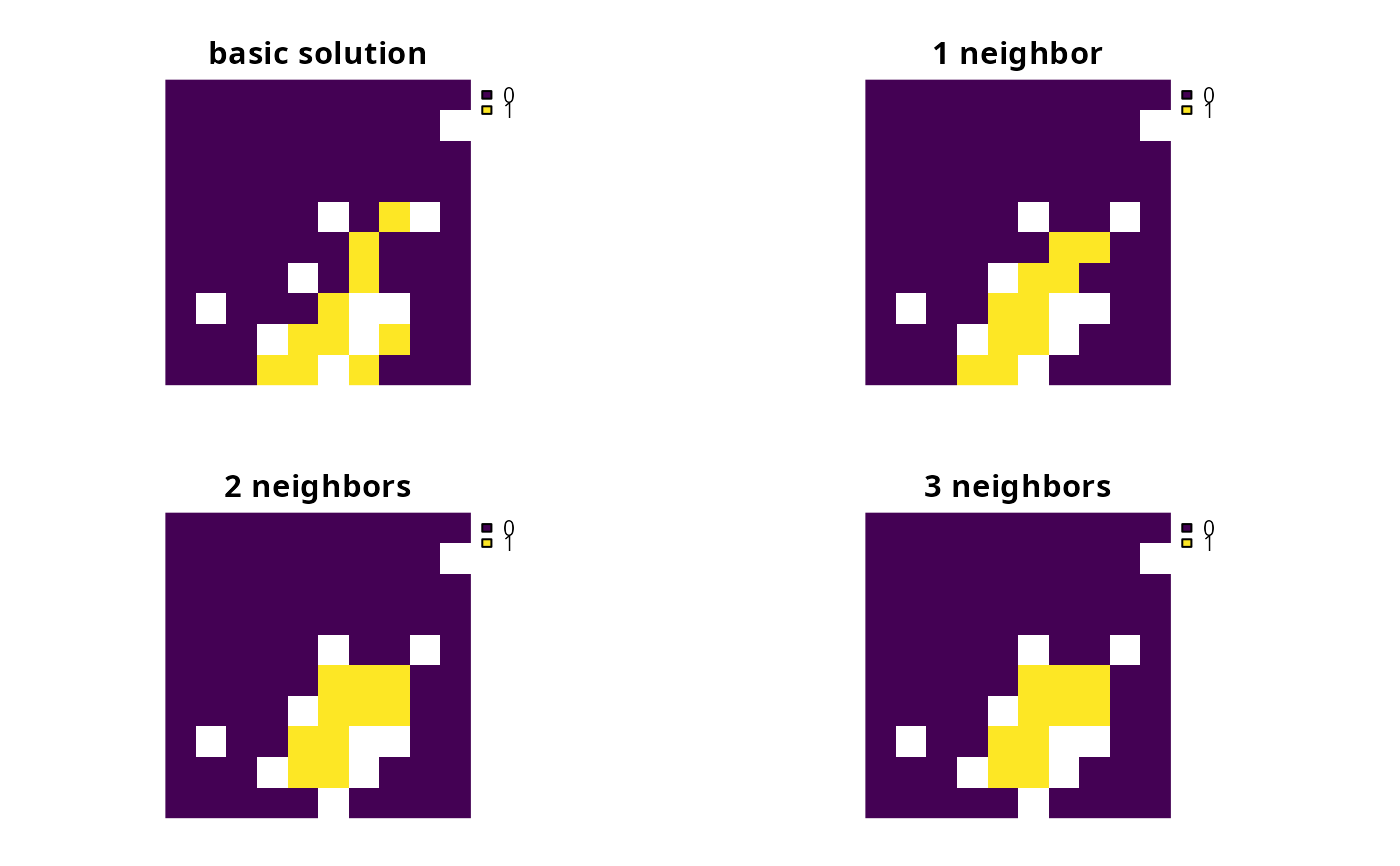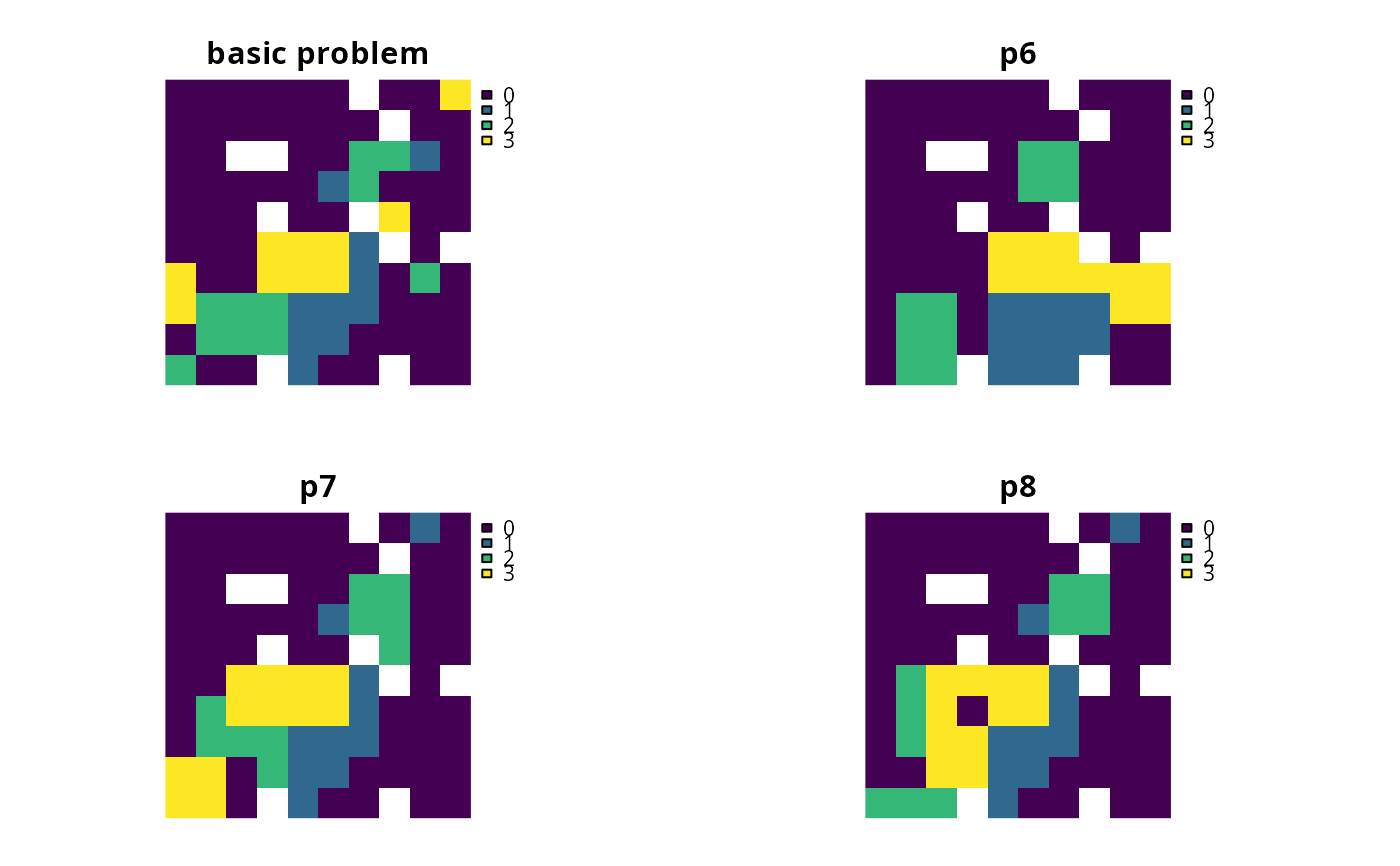Add constraints to a conservation planning problem() to ensure that all selected planning units in the solution have at least a certain number of neighbors that are also selected in the solution.

# S4 method for ConservationProblem,ANY,ANY,ANY

# S4 method for ConservationProblem,ANY,ANY,data.frame

# S4 method for ConservationProblem,ANY,ANY,matrix

# S4 method for ConservationProblem,ANY,ANY,array
add_neighbor_constraints(x, k, zones, data)

## Arguments

x

problem() (i.e., ConservationProblem) object.

k

integer minimum number of neighbors for selected planning units in the solution. For problems with multiple zones, the argument to k must have an element for each zone.

zones

matrix or Matrix object describing the neighborhood scheme for different zones. Each row and column corresponds to a different zone in the argument to x, and cell values must contain binary numeric values (i.e., one or zero) that indicate if neighboring planning units (as specified in the argument to data) should be considered neighbors if they are allocated to different zones. The cell values along the diagonal of the matrix indicate if planning units that are allocated to the same zone should be considered neighbors or not. The default argument to zones is an identity matrix (i.e., a matrix with ones along the matrix diagonal and zeros elsewhere), so that planning units are only considered neighbors if they are both allocated to the same zone.

data

NULL, matrix, Matrix, data.frame, or array object showing which planning units are neighbors with each other. The argument defaults to NULL which means that the neighborhood data is calculated automatically using the adjacency_matrix() function. See the Data format section for more information.

## Value

Object (i.e., ConservationProblem) with the constraints added to it.

## Details

This function uses neighborhood data identify solutions that surround planning units with a minimum number of neighbors. It was inspired by the mathematical formulations detailed in Billionnet (2013) and Beyer et al. (2016).

## Data format

The argument to data can be specified using the following formats:

data as a NULL value

neighborhood data should be calculated automatically using the adjacency_matrix() function. This is the default argument. Note that the neighborhood data must be manually defined using one of the other formats below when the planning unit data in the argument to x is not spatially referenced (e.g., in data.frame or numeric format).

data as a matrix/Matrix object

where rows and columns represent different planning units and the value of each cell indicates if the two planning units are neighbors or not. Cell values should be binary numeric values (i.e., one or zero). Cells that occur along the matrix diagonal have no effect on the solution at all because each planning unit cannot be a neighbor with itself.

data as a data.frame object

containing the fields (columns) "id1", "id2", and "boundary". Here, each row denotes the connectivity between two planning units following the Marxan format. The field boundary should contain binary numeric values that indicate if the two planning units specified in the fields "id1" and "id2" are neighbors or not. This data can be used to describe symmetric or asymmetric relationships between planning units. By default, input data is assumed to be symmetric unless asymmetric data is also included (e.g., if data is present for planning units 2 and 3, then the same amount of connectivity is expected for planning units 3 and 2, unless connectivity data is also provided for planning units 3 and 2). If the argument to x contains multiple zones, then the columns "zone1" and "zone2" can optionally be provided to manually specify if the neighborhood data pertain to specific zones. The fields "zone1" and "zone2" should contain the character names of the zones. If the columns "zone1" and "zone2" are present, then the argument to zones must be NULL.

data as an array object

containing four-dimensions where binary numeric values indicate if planning unit should be treated as being neighbors with every other planning unit when they are allocated to every combination of management zone. The first two dimensions (i.e., rows and columns) correspond to the planning units, and second two dimensions correspond to the management zones. For example, if the argument to data had a value of 1 at the index data[1, 2, 3, 4] this would indicate that planning unit 1 and planning unit 2 should be treated as neighbors when they are allocated to zones 3 and 4 respectively.

Other constraints: add_contiguity_constraints(), add_feature_contiguity_constraints(), add_linear_constraints(), add_locked_in_constraints(), add_locked_out_constraints(), add_mandatory_allocation_constraints,ConservationProblem-method, add_manual_bounded_constraints(), add_manual_locked_constraints()

## Examples

# load data
data(sim_pu_raster, sim_features, sim_pu_zones_stack, sim_features_zones)

# create minimal problem
p1 <- problem(sim_pu_raster, sim_features) %>%

# create problem with constraints that require 1 neighbor
# and neighbors are defined using a rook-style neighborhood

# create problem with constraints that require 2 neighbor
# and neighbors are defined using a rook-style neighborhood

# create problem with constraints that require 3 neighbor
# and neighbors are defined using a queen-style neighborhood
data = adjacency_matrix(sim_pu_raster, directions = 8))

# \dontrun{
# solve problems
s1 <- stack(list(solve(p1), solve(p2), solve(p3), solve(p4)))

# plot solutions
plot(s1, box = FALSE, axes = FALSE,
main = c("basic solution", "1 neighbor", "2 neighbors", "3 neighbors"))# }
# create minimal problem with multiple zones
p5 <- problem(sim_pu_zones_stack, sim_features_zones) %>%
add_relative_targets(matrix(0.1, ncol = 3, nrow = 5)) %>%

# create problem where selected planning units require at least 2 neighbors
# for each zone and planning units are only considered neighbors if they
# are allocated to the same zone
z6 <- diag(3)
print(z6)
#>      [,1] [,2] [,3]
#> [1,]    1    0    0
#> [2,]    0    1    0
#> [3,]    0    0    1
p6 <- p5 %>% add_neighbor_constraints(rep(2, 3), z6)

# create problem where the planning units in zone 1 don't explicitly require
# any neighbors, planning units in zone 2 require at least 1 neighbors, and
# planning units in zone 3 require at least 2 neighbors. As before, planning
# units are still only considered neighbors if they are allocated to the
# same zone
p7 <- p5 %>% add_neighbor_constraints(c(0, 1, 2), z6)

# create problem given the same constraints as outlined above, except
# that when determining which selected planning units are neighbors,
# planning units that are allocated to zone 1 and zone 2 can also treated
# as being neighbors with each other
z8 <- diag(3)
z8[1, 2] <- 1
z8[2, 1] <- 1
print(z8)
#>      [,1] [,2] [,3]
#> [1,]    1    1    0
#> [2,]    1    1    0
#> [3,]    0    0    1
p8 <- p5 %>% add_neighbor_constraints(c(0, 1, 2), z8)
# \dontrun{
# solve problems
s2 <- list(p5, p6, p7, p8)
s2 <- lapply(s2, solve)
s2 <- lapply(s2, category_layer)
s2 <- stack(s2)
names(s2) <- c("basic problem", "p6", "p7", "p8")

# plot solutions
plot(s2, main = names(s2), box = FALSE, axes = FALSE)# }